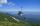# Pyramid

Pyramid has a base a = 5cm and height in v = 8 cm.

a) calculate angle between plane ABV and base plane
b) calculate angle between opposite side edges.

Result

α =  72.65 °
β =  33.22 °

#### Solution:Leave us a comment of example and its solution (i.e. if it is still somewhat unclear...):Be the first to comment!#### To solve this verbal math problem are needed these knowledge from mathematics:

See also our right triangle calculator. Most natural application of trigonometry and trigonometric functions is a calculation of the triangles. Common and less common calculations of different types of triangles offers our triangle calculator. Word trigonometry comes from Greek and literally means triangle calculation.

## Next similar examples:

1. FlowerbedFlowerbed has the shape of a truncated pyramid, the bottom edge of the base a = 10 m, the upper base b = 9 m. Deviation angle between edge and the base is alpha = 45°. What volume is needed to make this flowerbed? How many plants can be planted if 1 m2 =.
2. Angle of two linesThere is a regular quadrangular pyramid ABCDV; | AB | = 4 cm; height v = 6 cm. Determine the angles of lines AD and BV.
3. ReflectorCircular reflector throws light cone with a vertex angle 49° and is on 33 m height tower. The axis of the light beam has with the axis of the tower angle 30°. What is the maximum length of the illuminated horizontal plane?
4. MapleMaple peak is visible from a distance 3 m from the trunk from a height of 1.8 m at angle 62°. Determine the height of the maple.
5. BevelI have bevel in the ratio 1:6. What is the angle and how do I calculate it?On a straight stretch of road is marked 12 percent drop. What angle makes the direction of the road with the horizontal plane?
7. Reference angleFind the reference angle of each angle:
8. High wallI have a wall 2m high. I need a 15 degree angle (upward) to second wall 4 meters away. How high must the second wall?
9. TreeHow tall is the tree that observed in the visual angle of 52°? If I stand 5 m from the tree and eyes are two meters above the ground.
10. Map - climbOn the map of High Tatras in scale 1:11000 are cable car stations in the Tatranska Lomnica and in the Skalnate Pleso with distance 354.6 mm. Altitude of this stations are 949 m and 1760 m. What is average angle of climb of this cable car track?Average climb of the road is given by ratio 1:15. By what angle road average climb?We want to prove the sentence: If the natural number n is divisible by six, then n is divisible by three. From what assumption we started?If the average(arithmetic mean) of three numbers x,y,z is 50. What is the average of there numbers (3x +10), (3y +10), (3z+10) ?The class is 21 students. How many ways can choose two to examination?Solve the system of linear equations with three unknowns: A + B + C = 14 B - A - C = 4 2A - B + C = 0Determine the first nine elements of sequence if a10 = -1 and d = 4It is true that the lines that do not intersect are parallel?